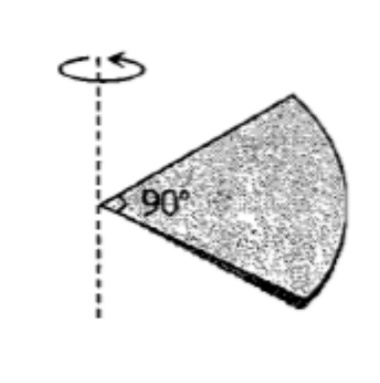One quarter sector is cut from a uniform circular disc of radius R.  This sector has mass M.  It is made to rotate about a line perpendicular to its plane and passing through the centre of the original disc.  Its moment of inertia about the axis of rotation is [IIT-JEE (Screening) 2001](1) $\frac{1}{2}M{R}^{2}$

(2) $\frac{1}{4}M{R}^{2}$

(3) $\frac{1}{8}M{R}^{2}$

(4) $\sqrt{2}M{R}^{2}$